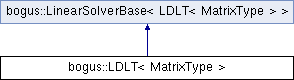So-Bogus A c++ sparse block matrix library aimed at Second Order cone problems
bogus::LDLT< MatrixType > Struct Template Reference

Base class for LDLT factorizations. More...

`#include <LinearSolverBase.hpp>`

Inheritance diagram for bogus::LDLT< MatrixType >:## Public Types

enum

typedef BlockTraits< typename
LinearSolverTraits< LDLT
< MatrixType > >::MatrixType >
UnderlyingBlockTraits

typedef
UnderlyingBlockTraits::Scalar
Scalar

## Public Member Functions

LinearSolverTraits< LDLT
< MatrixType > >::template
Result< RhsT >::Type
solve (const RhsT &rhs) const
Returns the solution x of the linear system M `*` x `=` `rhs`.

void solve (const RhsT &rhs, ResT &x) const
Finds the solution x of the linear system M `*` x `=` `b`.

const LDLT< MatrixType > & derived () const

LDLT< MatrixType > & derived ()

## Detailed Description

### template<typename MatrixType> struct bogus::LDLT< MatrixType >

Base class for LDLT factorizations.

The documentation for this struct was generated from the following file: